# Electricity

## Verification of Joule’s law

Videos:

c4science: Investigating Joule's Law

Simple Science and Maths: To Verify Joule's Law in the Lab

Apparatus and Method:

Verification of Joule’s law

Simulations: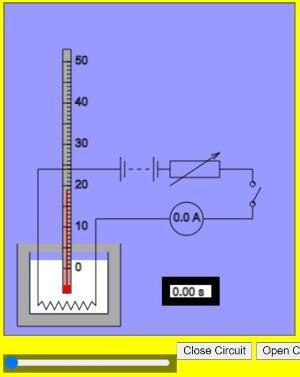Verification of Joule's Law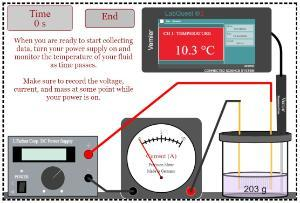Electrical Determination of Specific Heat Lab: While developed for the measurement of specific heat, this simulation also allows users to verify Joules Law.

## Measurement of the resistivity of the material of a wire

Videos:

c4science: The Resistivity of a Wire

Apparatus and Method:

Measurement of the resistivity of the material of a wire

Simulations: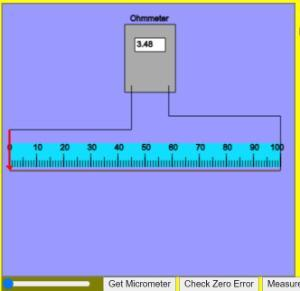Measurement of the resistivity of the material of a wireResistance of a Wire Challenge Lab

## To investigate the variation of the resistance of a metallic conductor with temperature

Videos:

Simple Science and Math: Variation of Resistance of a Metallic Conductor with Temperature

Apparatus and Method:

Variation of the resistance of a metallic conductor with temperature

Simulations:To Investigate the Variation of the Resistance of a Metallic Conductor with Temperature

## To investigate the variation of the resistance of a thermistor with temperature

Videos:

Simple Science and Maths: Variation of Resistance of a Thermistor with Temperature

Apparatus and Method:

To investigate the variation of the resistance of a thermistor with temperature

Simulations:To Investigate the Variation of the Resistance of a Thermistor with Temperature

## To investigate the variation of current (I) with pd (V) for: (a) metallic conductor. (b) filament bulb. (c) copper sulfate solution with copper electrodes. (d) semiconductor diode.

Videos:

Simple Science and Maths: Current vs Potential Difference for 4 Different Conductors

c4science: V-I Curve for a Filament Lamp

c4science: Graphing Current and P.D. for a Diode

Noel Cunningham: leaving cert diode

Apparatus and Method:

Simulations:

(A) Metallic Conductor: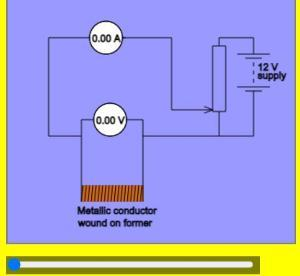To investigate the variation of current ( I ) with pd ( V ) for a metallic conductorOhm's law and resistance

(B) Filament Bulb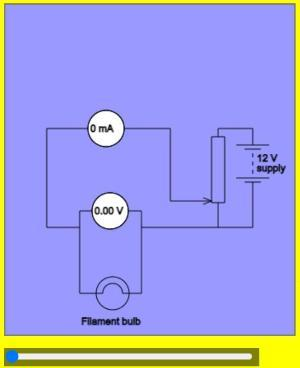To investigate the variation of current ( I ) with pd ( V ) for a filament bulb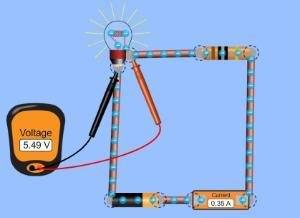Circuit Construction:  Use the "real bulb" option

(C) Copper Sulfate Solution with Copper Electrodes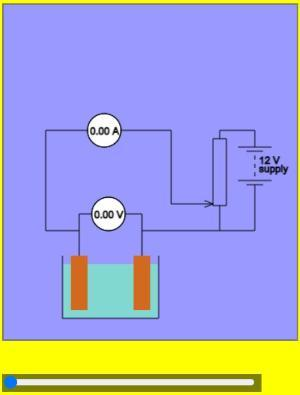To investigate the variation of current ( I ) with pd ( V ) for copper sulfate solution with copper electrodes

(D) Semiconductor Diode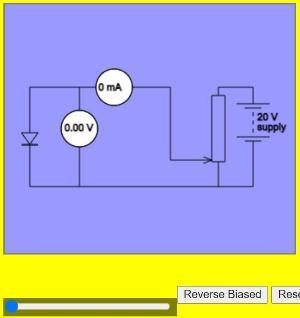To investigate the variation of current ( I ) with pd ( V ) for a semiconductor diode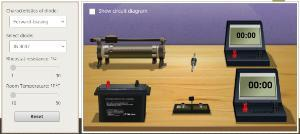Diode characteristics

## Scoil na Fisice

Room 213 (Physics Office), 2nd floor, Kane Science Building, University College Cork, Ireland.,

Top